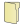Games
Problems
Go Pro!

# Proofs

Pro Problems > Math > Logic > Proofs

# Featured Pro ProblemsProve that there is no two digit number which is the product of its digitsThere are infinitely many angles between 0 and 90 degrees for which sine and cosine values are both rational. Create a proof.Use a proof by induction to predict the value of the sum of the first n perfect squares.Prove by contradiction that the harmonic mean of two positive numbers lies between the two numbersShow that the trig identity given is true for all non-negative integers n.Do a proof by induction to show what the sum of the first n positive integers is.Show that the expression given is an irrational expressionFind, with proof, the digits place of a product of consecutive integers# Full Directory Listing

Cubes and Nine, Ones Place Proof, Product of Digits, Rational Sine and Cosine, Squares Mod Four# Blogs on This SiteReviews and book lists - books we love!The site administrator fields questions from visitors.Like us on Facebook to get updates about new resources